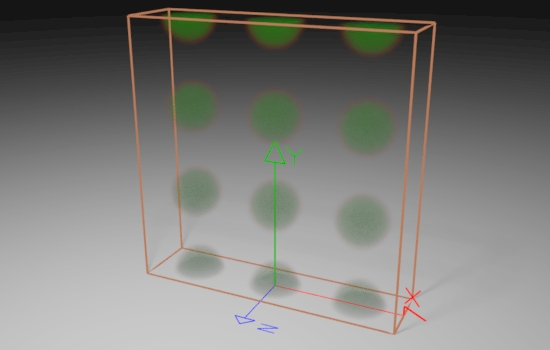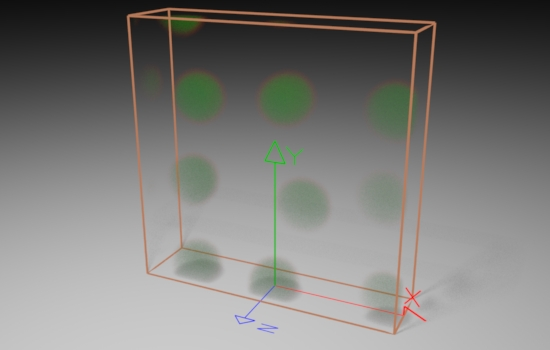### OSLCellnoise

#### Introduction

Pages 255 to 261 of "Advanced RenderMan" by Gritz and Apodaca provide an introduction to the RenderMan Shading Language function cellnoise(). This note attempts to explain, using OSL rather than RSL, how Gritz and Apodaca used cellnoise to create 3d textures.

#### Basic Code

The sample code used for this tutorial consists of a function and a shader given in listing 1. The function `dist2cell`() is almost identical to the code on pages 257 and 258 of the "Advanced RenderMan" book.

The three nested for-loops find the distance to the centers of cubes that form a 3x3x3 lattice of imaginary "cells" in the neighborhood of the shading point `P`. The function returns the distance to the jittered center of the nearest "cell". The shader uses the value returned by the function to determine if the shading point is within a user-defined `radius`.

Listing 1

 ```float dist2cell(point p, string spacename, float freq, float jitter) { point pp = transform(spacename, p) * freq; point thiscell = point(floor(pp) + 0.5, floor(pp) + 0.5, floor(pp) + 0.5); float dist2nearest = 1000; int i,j,k; for(i = -1; i <= 1; i += 1) for(j = -1; j <= 1; j += 1) for(k = -1; k <= 1; k += 1) { point testcell = thiscell + vector(i,j,k); point pos = testcell + (noise("cell", testcell) * jitter) - 0.5; float dist = distance(pos, pp); if(dist < dist2nearest) dist2nearest = dist; } return dist2nearest; } //------------------------------------------------------- shader cellular(float cellfreq = 5, float radius = 0.15, float blur = 0.1, float jitter = 1, color patcolor = color(1,0,1), color bakcolor = color(1,1,1), string spacename = "shader", output color resultRGB = 0, output float resultMask = 0, output float resultF = 0) { float d = dist2cell(P, spacename, cellfreq, jitter); resultF = 1 - smoothstep(radius, radius + blur, d); resultRGB = mix(bakcolor, patcolor, resultF); resultMask = (d <= radius) ? 1 : 0; } ```

So that the `cellular` shader can be used in several situations it has three outputs. In the renders shown below only resultRGB and resultF are used to "drive" the "diffuseColor" and "densityFloat" of a PxrVolume Bxdf.Figure 1 "cellular:jitter" <- 0Figure 2 "cellualar:jitter" <- PxrFractalFigure 2 "cellualar:jitter" <- PxrFractal "cellualar:radius" <- PxrFractal